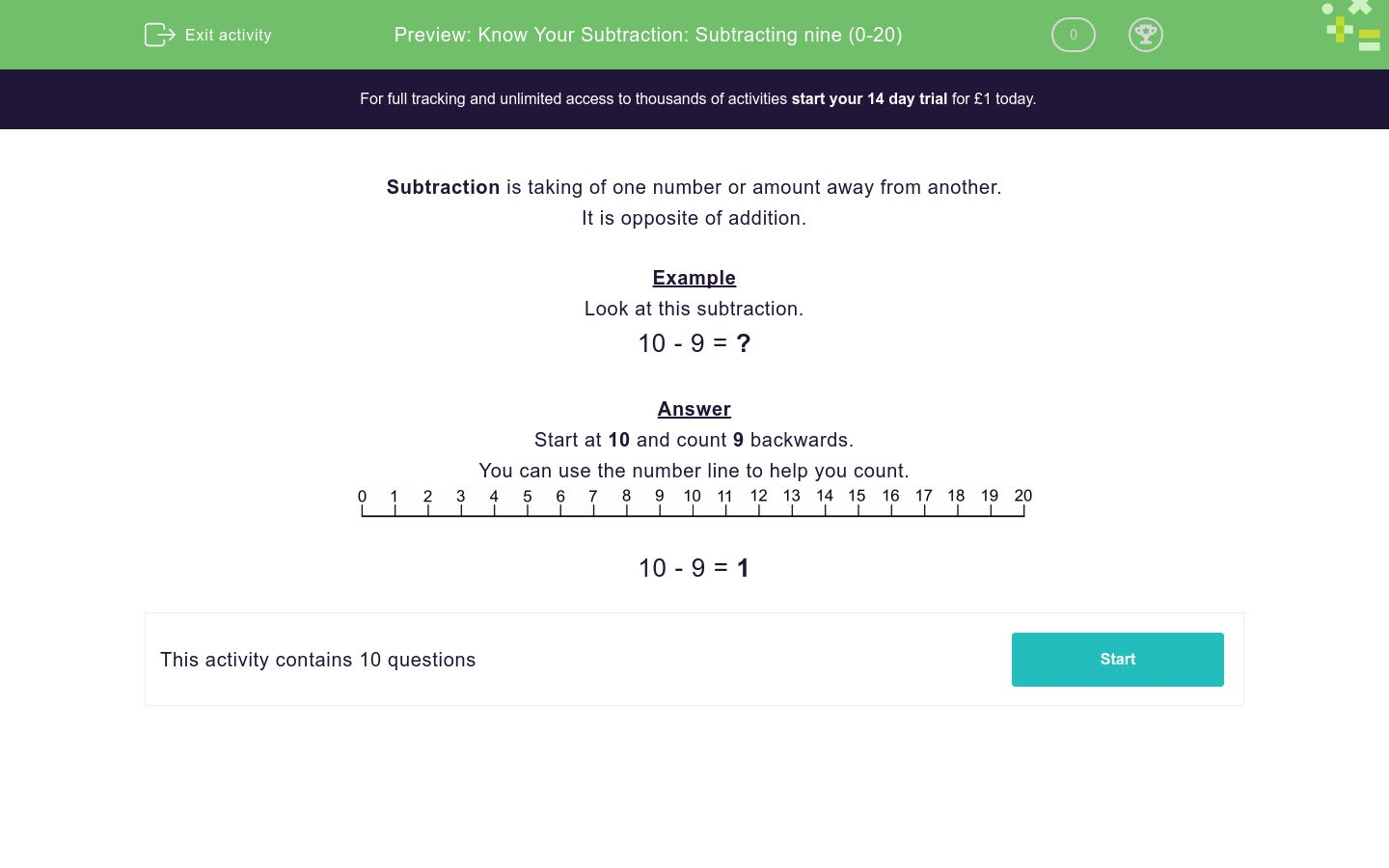# Know Your Subtraction: Subtracting nine (0-20)

In this worksheet, students subtract nine from a number between 0 and 20.Key stage:  KS 1

Curriculum topic:   Number: Addition and Subtraction

Curriculum subtopic:   Add and Subtract to 20

Difficulty level:### QUESTION 1 of 10

Subtraction is taking of one number or amount away from another.

Example

Look at this subtraction.

10 - 9 = ?

Start at 10 and count 9 backwards.10 - 9 = 1

Type the answer to this subtraction.14 - 9 = ?

Type the answer to this subtraction.15 - 9 = ?

Type the answer to this subtraction.13 - 9 = ?

Type the answer to this subtraction.18 - 9 = ?

Type the answer to this subtraction.16 - 9 = ?

Type the answer to this subtraction.12 - 9 = ?

Type the answer to this subtraction.11 - 9 = ?

Type the answer to this subtraction.17 - 9 = ?

Type the answer to this subtraction.20 - 9 = ?

Type the answer to this subtraction.10 - 9 = ?

• Question 1

Type the answer to this subtraction.14 - 9 = ?

5
EDDIE SAYS

14 − 9 = 5

Count back 9 from 14 to check.
• Question 2

Type the answer to this subtraction.15 - 9 = ?

6
EDDIE SAYS

15 − 9 = 6

To check, count back 9 from 15.
• Question 3

Type the answer to this subtraction.13 - 9 = ?

4
EDDIE SAYS

13 − 9 = 4

To check, count back 9 from 13.
• Question 4

Type the answer to this subtraction.18 - 9 = ?

9
EDDIE SAYS

18 − 9 = 9

To check, count back 9 from 18.
• Question 5

Type the answer to this subtraction.16 - 9 = ?

7
EDDIE SAYS

16 − 9 = 7

To check, count back 9 from 16.
• Question 6

Type the answer to this subtraction.12 - 9 = ?

3
EDDIE SAYS

12 − 9 = 3

To check, count back 9 from 12.
• Question 7

Type the answer to this subtraction.11 - 9 = ?

2
EDDIE SAYS

11 − 9 = 2

To check, count back 9 from 11.
• Question 8

Type the answer to this subtraction.17 - 9 = ?

8
EDDIE SAYS

17 − 9 = 8

To check, count back 9 from 17.
• Question 9

Type the answer to this subtraction.20 - 9 = ?

11
EDDIE SAYS

20 − 9 = 11

To check, count back 9 from 20.
• Question 10

Type the answer to this subtraction.10 - 9 = ?

1
EDDIE SAYS

10 − 9 = 1

To check, count back 9 from 10.
---- OR ----

Sign up for a £1 trial so you can track and measure your child's progress on this activity.

### What is EdPlace?

We're your National Curriculum aligned online education content provider helping each child succeed in English, maths and science from year 1 to GCSE. With an EdPlace account you’ll be able to track and measure progress, helping each child achieve their best. We build confidence and attainment by personalising each child’s learning at a level that suits them.

Get started